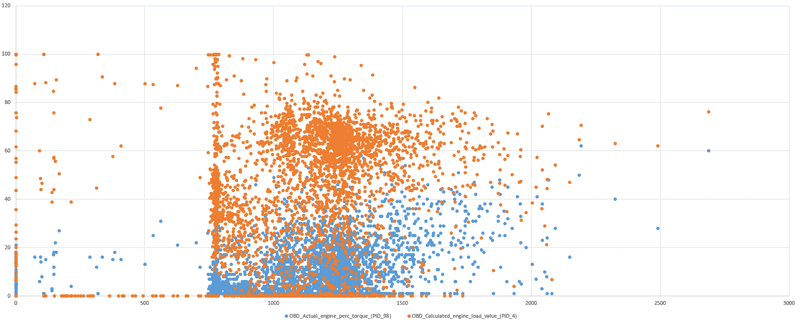# "Engine fuel consumption map" without the torque possible?

• Automotive
joker1319
Hello,
I am currently facing a problem.
I'm on a project and trying to create an "engine fuel consumption map (engine torque / engine speed)" among other things. Unfortunately, the engine torque is not measured in my measurements. More specifically, the "actual engine - percent torque (OBD PID 98)" outputs only "-125".

Therefore, I wonder if it is possible to derive the torque (approximately) with the following information I have:
• Velocity GPS km/h
• Velocity ECU km/h
• Engine Speed rpm
• Ambient Temperature degC
• Relative Humidity %
• Ambient Pressure kPa
• GPS Altitude m
• Altitude Amb, Pressure m
• Exhaust Temperature degC
• Exhaust Mass Flow g/s
• Exhaust Volume Flow (1atm 20degC) m3/s
• Coolant Temperature degC
• Intake Manif, Pressure Abs kPa
• Intake Manif, Pressure Rel kPa
• Intake Manif, Temperature degC
• Oil Temperature degC
• Throttle Position %
• Intake Mass Flow g/s
• OBD_Accelerator_Pedal_Position_D_(PID_73) %
• OBD_Ambient_air_temperature_(PID_70) °C
• OBD_Barometric_pressure_(PID_51) kPa
• OBD_Commanded_throttle_actuator_(PID_76) %
• OBD_Control_module_voltage_(PID_66) V
• OBD_Relative_throttle_position_(PID_69) %
• OBD_Run_time_since_engine_start_(PID_31) s
• va m2/s3
• va_pos m2/s3
• Acceleration m/s²
• m_CO2 g
• m_CO g
• m_NOx g
• n_PN -
• y_CO2 %
• y_CO ppm
• y_NO ppm
• y_NO2 ppm
• y_NOx ppm
• y_O2 %
• ndot_PN #/s
• mdot_CO2 g/s
• mdot_CO g/s
• mdot_NO g/s
• mdot_NO2 g/s
• mdot_NOx g/s
• mdot_O2 g/s

Some people claim that with the "OBD_Calculated_engine_load_value" somehow the engine torque can be derived. Unfortunately I have not yet found the formula of "Calculated_engine_load" which does not include the torque.

Mentor
Unfortunately I have not yet found the formula of "Calculated_engine_load" which does not include the torque.
Huh? If you want to calculate torque you need a formula with torque in it.

Engine torque * engine rpm * 2 pi/minute = engine mechanical power
The 2 pi/minute is converting rpm to an angular velocity. You have rpm, if you have the mechanical power of the engine you get torque.

joker1319
The problem is that the power of the car is not measured. It is only the power according to the vehicle documents known, but not the currentl power during the test.

Mentor

It is possible to estimate the power based on other values, like the speed of the car, the incline of the road, the density of air and some car-specific constants, but that will probably need experimental input.

joker1319
According to this..